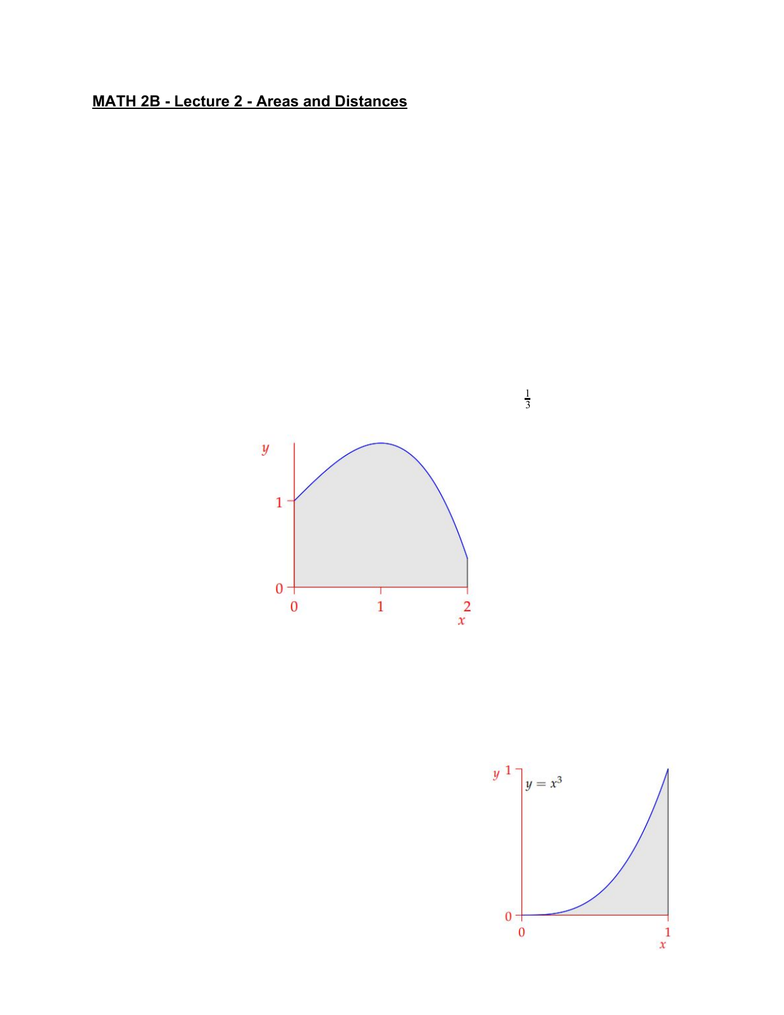Class Notes (1,100,000)
US (460,000)
UC-Irvine (10,000)
MATH (1,000)
MATH 2B (600)
Lecture 2

Department
Mathematics
Course Code
MATH 2B
Professor
ERJAEE, G.
Lecture
2

This preview shows half of the first page. to view the full 3 pages of the document.MATH 2B - Lecture 2 - Areas and Distances
Area of a Rectangle
The starting point of integral calculus is the problem of calculating area
. The
naÏve concept of area comes from the formula for the area of rectangle
:
Area = Length · Width
The area of a triangle is then immediately half that of a rectangle, and any shape
which may be subdivided into triangles may have its area computed.
Area Under the Curve
The primary question of integral calculus is of how to extend this idea to cover
shapes which cannot be built from triangles.
For example, below is the graph of the curve between 0 ≤ x ≤ 2;)xy = 1 + x3
13
how are we to compute the shaded area?
We approximate the area under the curve by rectangles and sum the areas of
these. If we take a larger number of rectangles, we will hopefully obtain a better
approximation to the desired area
Does this approximating process work for all functions?
Does it matter how
we choose the rectangles
The process of this is inefficient because a
human can’t evaluate the sum of 1500 area of
rectangles
Upper and Lower Bounds for Areas Example
The area under the curve between x = 0y=x3
and x = 1 is estimated using rectangles
Upper bound: n rectangles fitting just over curve